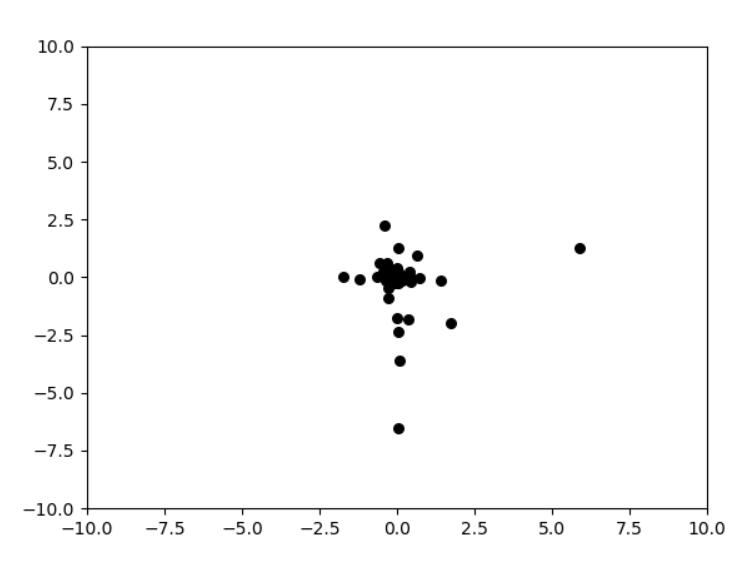# python粒子群算法的实现

http://blog.csdn.net/zuochao_2013/article/details/53431767?ref=myread

http://blog.csdn.net/chen_jp/article/details/7947059

## 算法介绍

粒子群算法(particle swarm optimization，PSO)由Kennedy和Eberhart在1995年提出，该算法对于Hepper的模拟鸟群(鱼群)的模型进行修正，以使粒子能够飞向解空间，并在最好解处降落，从而得到了粒子群优化算法。同遗传算法类似，也是一种基于群体叠代的，但并没有遗传算法用的交叉以及变异，而是粒子在解空间追随最优的粒子进行搜索

PSO的优势在于简单，容易实现，无需梯度信息，参数少，特别是其天然的实数编码特点特别适合于处理实优化问题。同时又有深刻的智能背景，既适合科学研究，又特别适合工程应用。
设想这样一个场景：一群鸟在随机的搜索食物。在这个区域里只有一块食物，所有的鸟都不知道食物在哪。但是它们知道自己当前的位置距离食物还有多远。那么找到食物的最优策略是什么？最简单有效的就是搜寻目前离食物最近的鸟的周围区域。

## 算法流程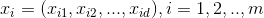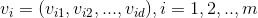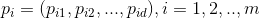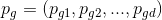PSO的关系公式

t时刻到t+1时刻的速度：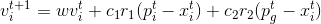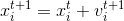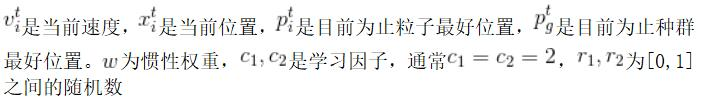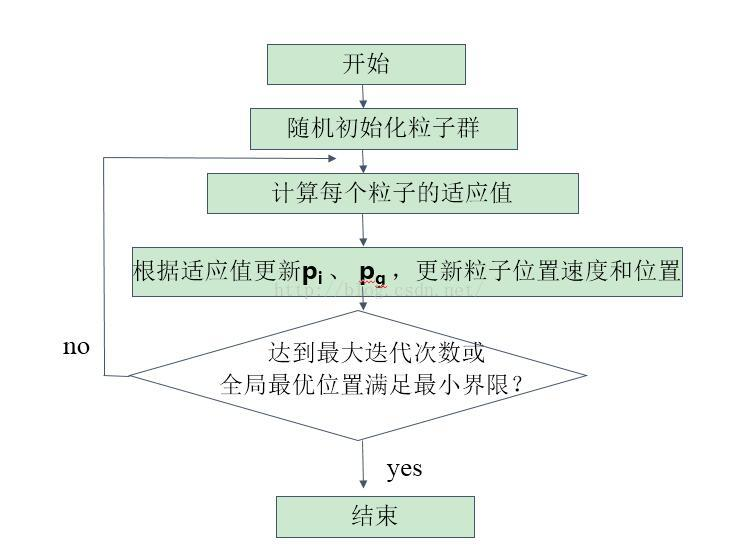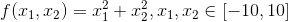在本例中，适应度就是函数值，适应度越小越好。在粒子群算法中，适应度不一定要越大越好，而是确定适应度的好坏，只需要根据是适应度好坏确定最佳位置。

在迭代过程中，会有粒子跑出范围，在这种情况下，一般不强行将粒子重新拉回到初始化解空间。因为即使粒子跑出空间，随着迭代的进行，如果在初始化空间内有更好的解存在，那么粒子也可以自行返回到初始化空间。研究表明，即使将初始化空间不设为问题的约束空间，粒子也可能找到最优解

import numpy as np
import matplotlib.pyplot as plt

class PSO(object):
def __init__(self, population_size, max_steps):
self.w = 0.6  # 惯性权重
self.c1 = self.c2 = 2
self.population_size = population_size  # 粒子群数量
self.dim = 2  # 搜索空间的维度
self.max_steps = max_steps  # 迭代次数
self.x_bound = [-10, 10]  # 解空间范围
self.x = np.random.uniform(self.x_bound, self.x_bound,
(self.population_size, self.dim))  # 初始化粒子群位置
self.v = np.random.rand(self.population_size, self.dim)  # 初始化粒子群速度
fitness = self.calculate_fitness(self.x)
self.p = self.x  # 个体的最佳位置
self.pg = self.x[np.argmin(fitness)]  # 全局最佳位置
self.individual_best_fitness = fitness  # 个体的最优适应度
self.global_best_fitness = np.min(fitness)  # 全局最佳适应度

def calculate_fitness(self, x):
return np.sum(np.square(x), axis=1)

def evolve(self):
fig = plt.figure()
for step in range(self.max_steps):
r1 = np.random.rand(self.population_size, self.dim)
r2 = np.random.rand(self.population_size, self.dim)
# 更新速度和权重
self.v = self.w*self.v+self.c1*r1*(self.p-self.x)+self.c2*r2*(self.pg-self.x)
self.x = self.v + self.x
plt.clf()
plt.scatter(self.x[:, 0], self.x[:, 1], s=30, color='k')
plt.xlim(self.x_bound, self.x_bound)
plt.ylim(self.x_bound, self.x_bound)
plt.pause(0.01)
fitness = self.calculate_fitness(self.x)
# 需要更新的个体
update_id = np.greater(self.individual_best_fitness, fitness)
self.p[update_id] = self.x[update_id]
self.individual_best_fitness[update_id] = fitness[update_id]
# 新一代出现了更小的fitness，所以更新全局最优fitness和位置
if np.min(fitness) < self.global_best_fitness:
self.pg = self.x[np.argmin(fitness)]
self.global_best_fitness = np.min(fitness)
print('best fitness: %.5f, mean fitness: %.5f' % (self.global_best_fitness, np.mean(fitness)))

pso = PSO(100, 100)
pso.evolve()
plt.show()